# Recursion Explained: How recursion works in Programming?

Recursion means “solving the problem via the solution of the smaller version of the same problem” or “defining a problem in terms of itself”. It is a widely used idea in programming to solve complex problems by breaking them down into simpler ones. In this blog, we’ll go over the basics of recursion and help you to refine an essential programming skill.

Recursion comes up in mathematics frequently, where there are many examples of expressions written in terms of themselves. Calculating the value of nth factorial and nth Fibonacci numbers is the best example of this. But recursion is just as much a programming concept!

• Finding the nth Factorial: n! = n * (n-1)!
• Finding the nth Fibonacci: F(n) = F(n-1) + F(n-2)

### Recursion in real life

Suppose you are standing in a long queue of people. How many people are directly behind you in the line?

Rules

• One person can see only the person standing directly in front and behind. So, one can't just look back and count.
• Each person is allowed to ask questions from the person standing in front or behind. How can we solve this problem recursively?

Solution

• You look behind and sees if there is a person there. If not, then you can return the answer "0". If there is a person, repeat step 1, and wait for a response from the person standing behind.
• Once a person receives a response, they add 1 for the person behind them, and they respond to the person that asked them or the person standing in front of them.

``````int peopleCount(Person curr)
{
if (noOneBehind(currPerson))
{
return 0
}
else
{
Person personBehind = curr.getBehind()
return peopleCount(personBehind) + 1
}
}
``````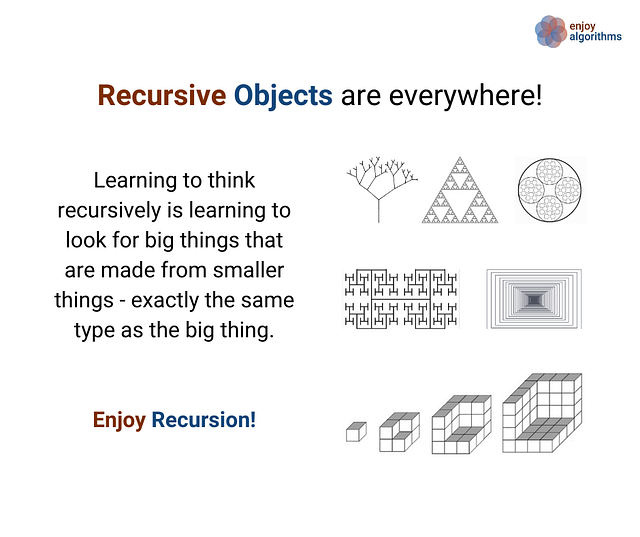### Recursion in programming

In programming terms, recursion is a function calling itself until a “base condition” is true to produce the correct output.

``````void function()
{
base case
.. .. ...
function() //recursive call
.. .. ...
}

int main()
{
... .. ...
function()
... .. ...
}
``````

In other words — To solve a problem, We solve a subproblem that is a smaller instance of the same problem and then use the solution to that smaller instance to solve the original problem. For a recursive algorithm to work, the smaller subproblems must eventually arrive at the base case.

The recursive algorithm has two parts:

1. Base Case: A terminating condition where a function can immediately return the result. This is the smallest version of the problem for which we already know the solution.
2. Recursive Structure: Solution to a problem via the solution of its smaller sub-problems i.e. same problem but for smaller input size. Here function must call itself to break the current problem down to a simpler level.

### A strategy for recursive problem solving

Steps to follow

• Define Base Case: Think of the smallest version of the problem and write down the solution.
• Define Recursive Structure: Now, assume we have a function to solve a problem of the given input size. How could we use it on a smaller input size and use the answer to solve a bigger size?
• Combine the base case and the recursive structure

Things to keep in mind

• Our code must cover all valid instances with smaller input sizes.
• We must have a base case that makes no recursive calls.
• When we make a recursive call, it should call a smaller instance and progress towards the base case.
• When we have a correct recursive structure and base case, then recursion would solve the problem for us. This is a "recursive leap of faith" where we should not worry about intermediate steps of the recursive calls.

### Basic examples of recursive functions

• Calculate the sum of two numbers using recursion

``````sum(x, y)
= x, if(y == 0)
= 1 + sum (x, y-1), if(y > 0)
``````
• Calculate the product of two numbers using recursion

``````product(x, y)
= 0, if(y == 0)
= sum (x, product(x, y-1), if(y > 0)
``````
• Calculate power of two numbers using recursion

``````power(x, y)
= 1, if(y == 0)
= product (x, power(x, y-1), if(y > 0)
``````

### Understanding recursion via finding nth Factorial

Factorial of a non-negative integer is a multiplication of all integers smaller than or equal to n. For example. the factorial of 5 is 1*2*3*4*5 = 120

Recursive structure

According to the mathematical definition of the factorial of n, we can write

``````n!
= n *  (n-1) * (n-2) *….* 2 * 1
= n * (n-1)!

=> nth factorial = n * (n-1)th factorial
``````

If we calculate the value of the (n-1)th factorial, we can easily calculate the value of the nth factorial. It means we can solve the problem of input size n with its smaller subproblem of the input size (n-1). In other words, we can solve this problem by using the idea of recursion.

Suppose the function fact(n) and fact(n-1) return the value of the nth and (n-1)th factorial, respectively, then we can write the following recursive structure.

``````fact(n) = n * fact(n-1)
``````

Base case

In every recursive solution, there must be a terminating condition or base case where our recursion will directly give you results without breaking it again into the sub-problem. If we observe the above recursive structure, then we find the following chain of recursive calls :

``````fact(n)
= n * fact(n-1)
= n * (n-1) * fact(n-2)
... and so on
= n * (n-1) * (n-2) * ... * 4 * 3 * 2 * fact(1)
= n * (n-1) * (n-2) * ... * 4 * 3 * 2 * 1 * fact(0)
``````

The factorial of a negative number is not defined, so fact(0) is the smallest version of the factorial problem where our recursion will terminate. So n = 0 is the base case that will return the value 1.

Recursive pseudocode of nth Factorial

``````int fact(int n)
{
if(n == 0)
return 1
return n * fact(n-1)
}
``````

### How does recursion works in the background?

If we draw the flow of recursion of the above pseudo-code, one can find this pattern: we are calling fact(0) last, but it is returning the value first. Similarly, we are calling fact(n) first, but it is returning the value last. Did you find some Last In First Out (LIFO) orders for recursive calls and return values? Yes, you got it right! Behind the scene, the compiler uses a stack data structure to simulate recursion and deliver the correct output. We call this stack: Call Stack!

• Order of recursive calls: larger problem to smaller problem

fact(n)-> fact(n-1)...-> fact(i)->...-> fact(1)-> fact(0)

• Order of return values: smaller problem to larger problem

fact(0)-> fact(1)...-> fact(i)->...-> fact(n-1)-> fact(n)

How the idea of call stack work in recursion?

• The information about the execution of a recursive function is stored in the call stack. It contains details about the execution: the current state of the control flow, local variables, and other internal information. When any function is called from main(), the memory is allocated to it on the stack.
• During the recursion, when the function calls the same function for a smaller input size, memory is allocated to it, and it goes on the top of the call stack.
• The memory for a called function is allocated on top of memory allocated to the calling function, and a different copy of local variables is created for each function call.
• When the base case is reached, the function returns its value to the function by whom it is called, and memory is de-allocated, and the process continues.

Recursion visualization for calculating fact(5)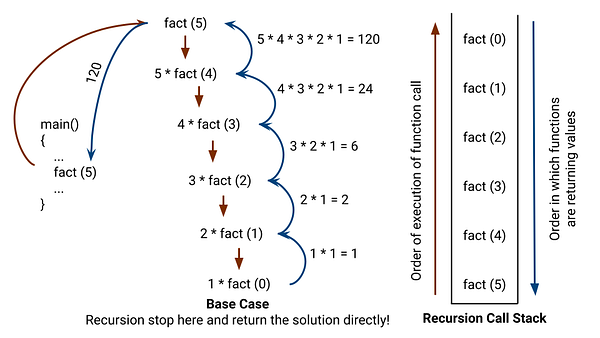Recursion visualization for calculating fact(3)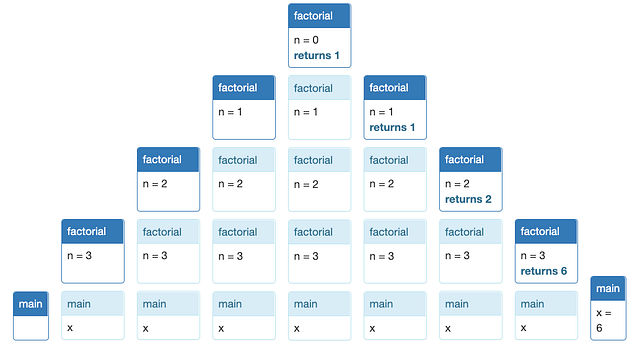Image Source: mit.edu

### Famous recursive algorithms

Reverse an array

• Recursive structure: reverse (A[], l, r) = swap(A[l], A[r]) + reverse(A, l+1, r-1)
• Base case: if (l >= r) then return
• Recurrence relation: T(n) = T(n-2) + c, Time complexity = O(n)

Finding the GCD of two numbers

• Recursive structure: GCD(a, b) = GCD(b, a mod b), here assuming that a > b
• Base case: GCD(a, 0) = a
• Recurrence relation: T(n) = T(n/d) + c, where d is a decreasing factor, Time complexity = O(log b)

Finding the nth Fibonacci

• Recursive structure: fib(n) = fib(n-1) + fib(n-2)
• Base case: We have 2 base cases: fib(0) = 0 and fib(1) = 1
• Recurrence relation: T(n) = T(n-1) + T(n-2) + C, Time complexity = O(2^n)

Tower of Hanoi problem

• Recursive structure: We move a stack of n disks from peg X to Y using peg Z

• Move top n-1 disks from X to Z
• Move bottom disk from X to Y
• Move top n-1 disks from Z peg to Y
• Base case: If n = 1, move disk from X to Y
• Recurrence relation: T(n) = 2 T(n-1) + C, Time complexity = O(2^n)

Binary search

• Recursive structure: binarySearch(A[], l, r, k)

• if A[mid] = k, return mid
• if (A[mid] > k), binarySearch(A[], l, mid-1, k)
• if (A[mid] < k), binarySearch(A[], mid+1, r, k)
• Base case: If (l > r) then return -1
• Recurrence relation: T(n) = T(n/2) + C, Time complexity = O(log n)

Merge sort

• Recursive structure: mergeSort (A[], l, r)

• mergeSort(A, l, mid)
• mergeSort(A, mid+1, r)
• merge(A, l, mid, r)
• Base case: if (l == r) then return. This is a case of a single-element array.
• Recurrence relation: T(n) = 2 T(n/2) + cn, Time complexity = O(n log n)

Quick sort

• Recursive structure: quickSort(A[], l, r)

• pivot = partition(A, l, r)
• quickSort(A, l, pivot - 1)
• quickSort(A, pivot + 1, r)
• Base case: if (l >= r) then return (Think!)
• Recurrence relation: T(n) = Sum (i = 0 to n-1) [T(i) + T(n-i-1)]/ n, Time complexity = O(nlogn) [Average case analysis]

• return remaining
• Recurrence relation: T(n) = T(n-1) + c, Time complexity = O(n)

Post-order traversal of the binary tree

• Recursive structure: postorder(root)

• postorder(root->left)
• postorder(root->right)
• Visit the root
• Base case: if(root == NULL), then return
• Recurrence relation: T(n) = T(n-1) + c, Time complexity = O(n)

Print all permutation of a given string

• Recursive structure: permute(S[], l, r)

``````for(i = l to r)
{
swap(S[l], S[i])
permute(S, l+1, r)
swap(S[l], S[i])
}
``````
• Base case: if(l == r) then print(A)
• Recurrence relation: T(n) = sum (i = 0 to n-1) [T(n-i) + c], Time complexity = O(n!)

### Iteration vs Recursion

Mode of implementation

• Iteration - Implemented using loops and defined by the value of the control variables.
• Recursion - Implemented using function calls and defined by the value of the function parameters stored in the call stack.

Nature of code error

• Iteration - An infinite loop occurs due to a mistake in iterator assignment or increment, or in the terminating condition or wrong terminating condition. This will consume system resources like processor time or memory and stop the program execution.
• Recursion - Infinite recursion may occur due to the absence of a base case or the wrong base case. It will cause a stack overflow scenario which may lead to a CPU crash.

Analysis of code

• Iteration - Most of the time analysis of the Iterative code is simple and intuitive.
• Recursion - Analysis of recursive code is difficult most of the time due to the complex recurrence relations.

Code execution

• Iteration - Iteration does not involve any such overhead.
• Recursion - Recursion is usually slower because it has a large amount of overhead of function calls. Function calls must be stored in a stack to allow the return back to the caller functions.

Code size

• Iteration - Iterative code size is usually bigger.
• Recursion - Recursion decreases the size of the code.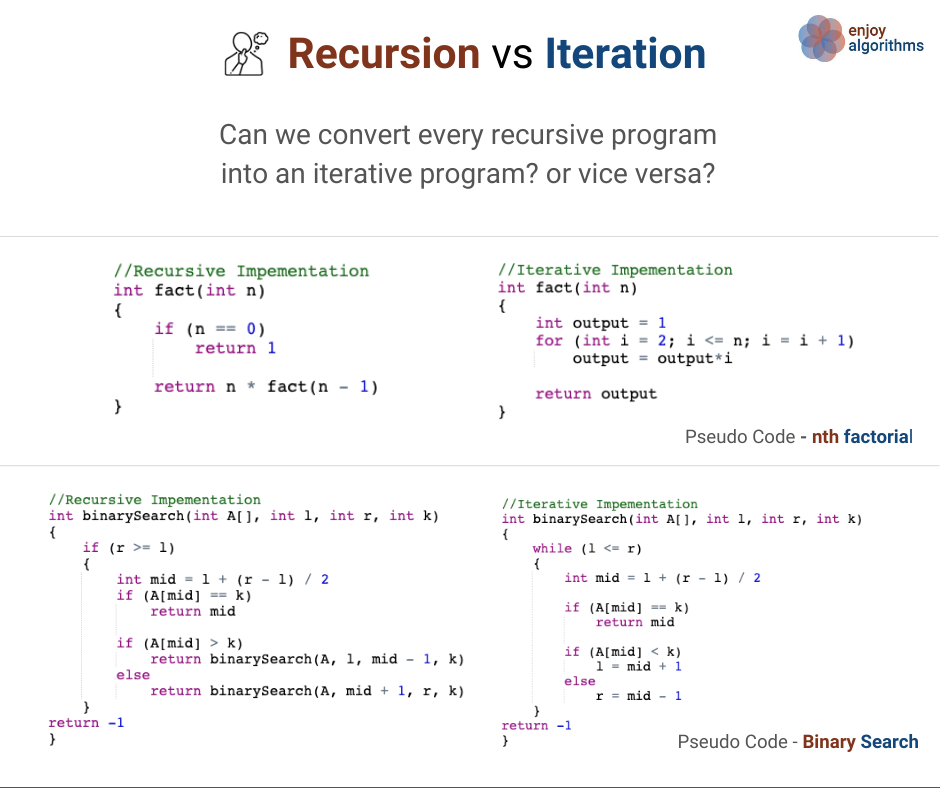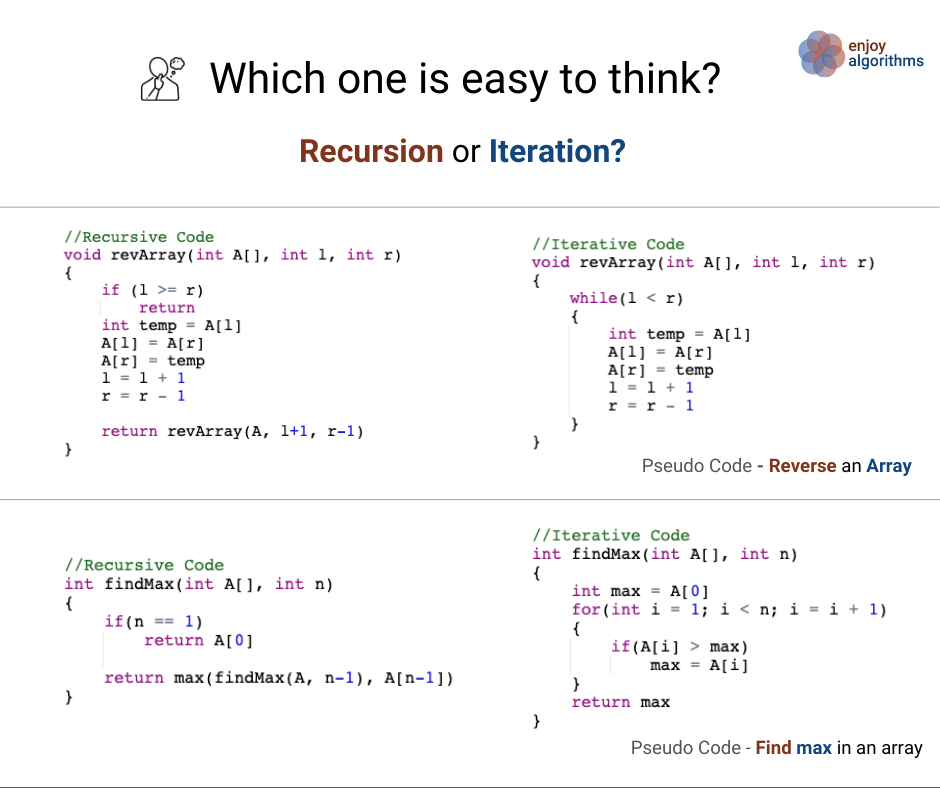### Stack overflow error in recursion

When we call a recursive function, the return address and arguments are pushed onto the call stack. The stack is finite, so if the recursion is too deep, you'll eventually run out of stack space. This is also called the stack overflow in recursion. In some situations, if the recursive function is tail-recursive, some compilers might optimize the recursive call away by turning it into a jump. Popular reasons for stack overflow error:

• The recursive function won't have any base case.
• A recursive function call with an incorrect base case

### Common mistakes in recursive implementations

Here are two common ways that a recursive implementation can go wrong:

• The base case is missing entirely, or the problem needs more than one base case, but not all the base cases are covered.
• The recursive step doesn’t reduce to a smaller subproblem, so the recursion doesn’t converge.

Look for these when you’re debugging. On the bright side, an infinite loop in an iterative implementation usually becomes a Stack Overflow Error in a recursive implementation. A buggy recursive program fails faster.

### Application of recursion in problem-solving

• Divide and Conquer Approach, Decrease and Conquer
• Searching and sorting algorithms: Binary search, Merge sort, Quicksort, etc.
• Problem-solving using recursive tree traversals
• Problem-solving using DFS in a graph
• Solving Dynamic Programming problems
• Problem-solving using Backtracking
• Designing approximation algorithms

### Concepts to explore further in recursion

• Analysis of recursion
• Types of recursion
• Tail recursion and recursive optimizations
• The idea of functional programming

### Content references

Enjoy learning, Enjoy coding, Enjoy algorithms!

Subscribe to get well-designed content on data structures and algorithms, machine learning, system design, oops, and mathematics. enjoy learning!

### We Welcome Doubts and Feedback!